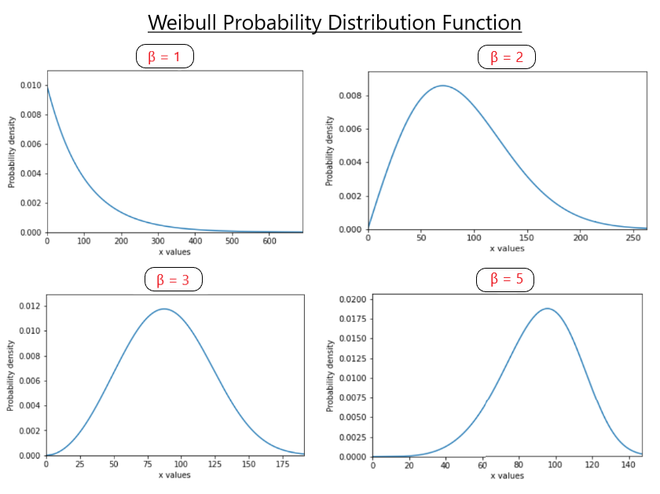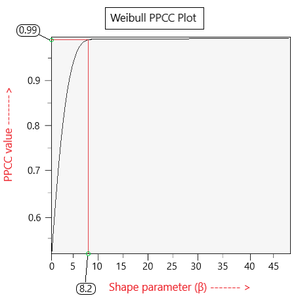# Weibull PPCC plot

• Last Updated : 16 Mar, 2021

Prerequisites: Weibull Plot

There are many probability distributions that are not single, but a family of distributions. This is due to the fact that they have multiple shape parameters. Weibull distribution is also family of distributions that has a variety of shapes based on the value of its shape parameter.

Attention reader! Don’t stop learning now. Get hold of all the important Machine Learning Concepts with the Machine Learning Foundation Course at a student-friendly price and become industry ready.

Shape parameter: It is a value that determines the shape that a distribution takes. For example, the weibull distribution has a shape parameter β. (or γ in some places) The shape of the plot or the distribution changes on varying the values of α. This shape parameter is also known as the weibull slope as any changes done in α changes the slope of the plot as well. [See Fig 1]Fig 1: Weibull PDF on varying values of β

Probability plot cost coefficient (PPCC):

The PPCC is a graphical technique that helps in identifying a good value of the shape parameter of a distributional family such that it best fits the given data set. In statistical terms, it determines the goodness of fit of a distribution on a data set. Its value ranges from 0 to 1.

Structure: The ppcc plot has the following parameters on the axes:

• x-axis: shape parameter value. (β in case of weibull)
y-axis: ppcc value corresponding to the shape parameter.

Weibull PPCC plot:

In plots such as weibull, the PPCC plot helps in identifying the best shape parameter (β) that gives the best fit weibull distribution over the dataset. Take a look at Fig 2.Fig 2: Sample Weibull plot

Intuition:

The appropriate value of the shape parameter (β) is determined by comparing the PPCC value associated to it. The value of β that corresponds to the highest PPCC value (nearing to 1) is selected as the shape parameter.

However, when choosing from multiple distributions, we do not always go for the one with the highest PPCC value. We take into account the complexity of the distribution as well. Say, if a distribution is simpler but with a slightly less PPCC value compared to that of a complex distribution family, then we will opt for the simpler one.

Importance: Many times during a weibull analysis, an assumption is made that the given data follows a Weibull distribution. Hence, it becomes very important to verify this assumption and, if verified, find good estimates of the Weibull parameters. In such cases, Weibull PPCC plots play a huge role in determining the optimum values of the parameters so that we can get good results from our analysis.

My Personal Notes arrow_drop_up